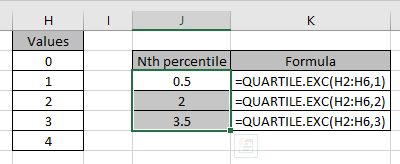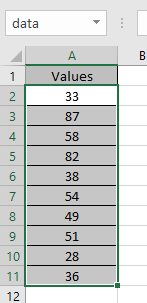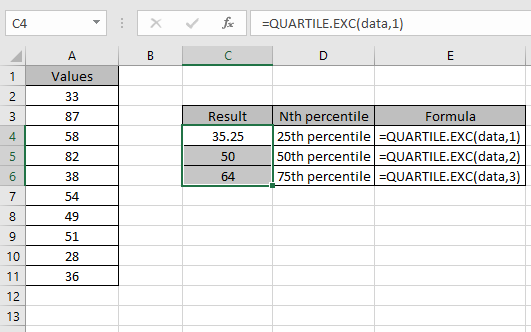# How to use the QUARTILE.EXC Function in Excel

QUARTILE.EXC function returns the nth percentile of the range of values. From Excel 2010 QUARTILE.EXC function is preferred

It returns 1/4th QUARTILE.EXC value, 1/2th QUARTILE.EXC value & 3/4th value maximum value from the array of numbers.
Syntax:

=QUARTILE.EXC(array, quart)

Array : list of numbers
Quart : select a number to get the nth percentile you wish

 Quart Nth percentile 1 25th percentile 2 50th percentile 3 75th percentile

Let’s run some tests on some numbers using the function.

The nth percentile value for the range of values : 0,1,2,3,4
Use the formula:
Let’s run some tests on some numbers using the function.

The nth percentile value for the range of values : 0,1,2,3,4
Use the formula:

=QUARTILE.EXC(H2:H6,1)

Replace the quart_num with other numbers to get different percentile values from the array values.As you can see,
25th percentile is 0.5, 50th percentile is 2 & 75th percentile for the array will be 3.5.

Now we wish to find the QUARTILE.EXC of the data setThe data name is defined for the values A2:A11.
Use the formula

=QUARTILE.EXC (data, 1)As you can see data can be extracted from the values of array using Excel QUARTILE.EXC function.

Notes:

1. QUARTILE.EXC returns the #NUM! error value.
• If the array input is empty.
• If quart is less than 1 or more than 3
2. If quart number is not an integer, it gets truncated.
3. MEDIAN value can be extracted from the same value as QUARTILE.EXC when quart is equal to 2.

Hope you understood how to use QUARTILE.EXC function and referring cell in Excel. Explore more articles on Excel functions here. Please feel free to state your query or feedback for the above article.

Related Articles:

How to use the QUARTILE.INC Function in Excel

How to use the Excel Quartile function

Popular Articles:

50 Excel Shortcuts to Increase Your Productivity

How to use the VLOOKUP Function in Excel

How to use the COUNTIF in Excel 2016

How to use the SUMIF Function in Excel

Terms and Conditions of use

The applications/code on this site are distributed as is and without warranties or liability. In no event shall the owner of the copyrights, or the authors of the applications/code be liable for any loss of profit, any problems or any damage resulting from the use or evaluation of the applications/code.